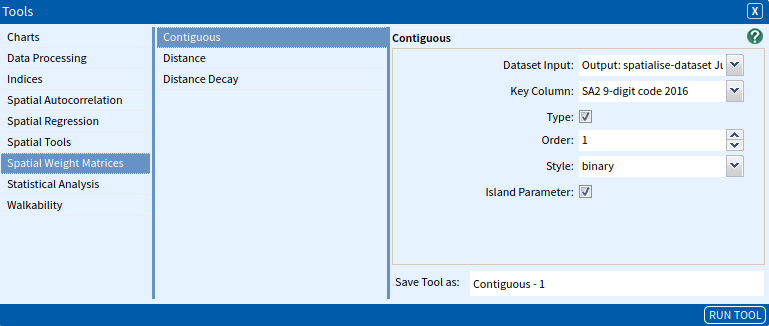# Getis-Ord Global G

The focus of the family of G statistics derived by Getis and Ord (1992; Ord & Getis, 1995) is on spatial autocorrelation at the local level and can be calculated with the Getis-Ord Local G tool. However, they did include a global measure of spatial autocorrelation. The Global G is defined as (Getis & Ord, 1992, p. 194)

$G(d) = {{\sum\nolimits_{i=1}^n\sum\nolimits_{j=1}^n w_{ij}(d)X_{i}X_{j}}\over{\sum\nolimits_{i=1}^n\sum\nolimits_{j=1}^n X_{i}X_{j}}}$

Following the usage recommendation (Bivand et al., 2019, p. 39) all spatial weight matrices will be converted to binary weights (style “B”) if they are not already.

The $G(d)$ statistic is concerned with the overall concentration or lack of concentration in all pairs that are neighbours given the definition of neighbouring areas. The variable must contain only positive values to be used.

### SET UP

To compute the Getis-Ord Global G statistic, we will look at socio-economic data in Melbourne to examine the extent of spatial-autocorrelation.

• Select Melbourne Inner SA4 (Australia → Victoria → Greater Melbourne → Melbourne – Inner) as your area.
• Select ABS – Socio-Economic Indexes for Areas (SEIFA) – The Index of Relative Socio-economic Advantage and Disadvantage (SA2) 2016 as your dataset, selecting all variables
• Spatialise the dataset using the Spatialise Aggregated Dataset tool or from the spanner tool on the dataset.• Generate a Contiguous Spatial Weight Matrix for the spatialised dataset, using 1st order Queen contiguity and set for binary weights.### Inputs

Once you have done this, open the Getis-Ord Global G tool (Tools → Spatial Autocorrelation → Getis-Ord Global G) and enter the following parameters:

• Dataset Input: The dataset that contains the variable(s) to be tested. Select the spatialised dataset.
• Spatial Weights Matrix: The spatial weight matrix to be used. Select the spatial weights matrix dataset.
• Key Column: The unique identifier for each row. Select SA2 9-digit code 2016.
• Variable: The variable(s) to be tested. Select IRSAD Score.
• Alternative Hypothesis: Indicates the alternative hypothesis; this can be two.sided, greater. Select two.sided.Once you have entered the parameters, click Run Tool to execute the tool.

### Outputs

Once your tool has run, click the Display Output button on the pop-up dialogue box. This will open a text editor which should look something like the image below.These outputs are:

• Global G with the corresponding standard deviate and p-value.
• Global G with its expectation and variance.
• The alternative hypothesis.
Bivand, R., Altman, M., Anselin, L., Assunção, R., Berke, O., Bernat, A., & Blanchet, G. (2019). Spatial dependence: Weighting schemes, statistics and models.
Getis, A., & Ord, K. (1992). The Analysis of Spatial Association by Use of Distance Statistics. Geographical Analysis, 24, 189–206.
Ord, J. K., & Getis, A. (1995). Local spatial autocorrelation statistics: Distributional issues and an application. Geographical Analysis, 27(4), 286–306.

### Looking for Spatial Data?

You can browse the AURIN Data Discovery:### How can you Create Impact?